# Augment Pixel Labels for Semantic Segmentation

This example shows how to perform common kinds of image and pixel label augmentation as part of semantic segmentation workflows.

Semantic segmentation training data consists of images represented by numeric matrices and pixel label images represented by categorical matrices. When you augment training data, you must apply identical transformations to the image and associated pixel labels. This example demonstrates three common types of transformations:

The example then shows how to apply augmentation to semantic segmentation training data in datastores using a combination of multiple types of transformations.

You can use augmented training data to train a network. For an example showing how to train a semantic segmentation network, see Semantic Segmentation Using Deep Learning (Computer Vision Toolbox).

To demonstrate the effects of the different types of augmentation, each transformation in this example uses the same input image and pixel label image.

Read a sample image.

```filenameImage = 'kobi.png'; I = imread(filenameImage);```

Read the pixel label image. The image has two classes.

```filenameLabels = 'kobiPixelLabeled.png'; L = imread(filenameLabels); classes = ["floor","dog"]; ids = [1 2];```

Convert the pixel label image to the categorical data type.

`C = categorical(L,ids,classes);`

Display the labels over the image by using the `labeloverlay` function. Pixels with the label "floor" have a blue tint and pixels with the label "dog" have a cyan tint.

```B = labeloverlay(I,C); imshow(B) title('Original Image and Pixel Labels')```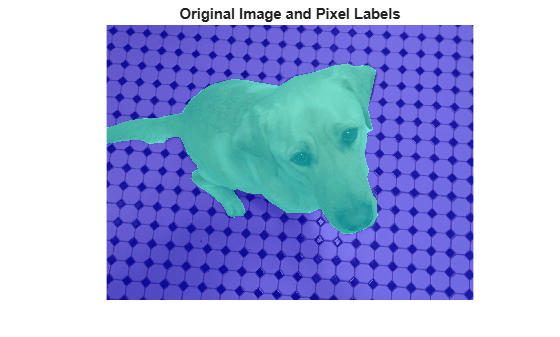### Resize Image and Pixel Labels

You can resize numeric and categorical images by using the `imresize` function. Resize the image and the pixel label image to the same size, and display the labels over the image.

```targetSize = [300 300]; resizedI = imresize(I,targetSize); resizedC = imresize(C,targetSize);```

Display the resized labels over the resized image.

```B = labeloverlay(resizedI,resizedC); imshow(B) title('Resized Image and Pixel Labels')```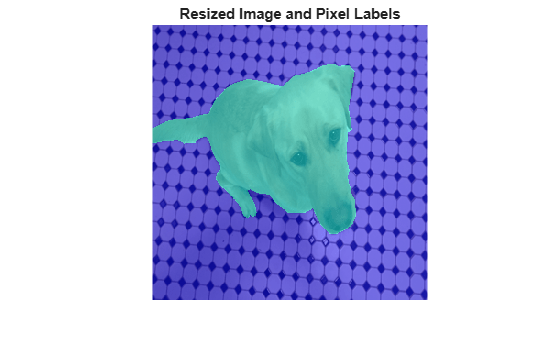### Crop Image and Pixel Labels

Cropping is a common preprocessing step to make the data match the input size of the network. To create output images of a desired size, first specify the size and position of the crop window by using the `randomWindow2d` (Image Processing Toolbox) and `centerCropWindow2d` (Image Processing Toolbox) functions. Make sure you select a cropping window that includes the desired content in the image. Then, crop the image and pixel label image to the same window by using `imcrop`.

Specify the desired size of the cropped region as a two-element vector of the form [height, width].

`targetSize = [300 300];`

Crop the image to the target size from the center of the image.

```win = centerCropWindow2d(size(I),targetSize); croppedI = imcrop(I,win); croppedC = imcrop(C,win);```

Display the cropped labels over the cropped image.

```B = labeloverlay(croppedI,croppedC); imshow(B) title('Center Cropped Image and Pixel Labels')```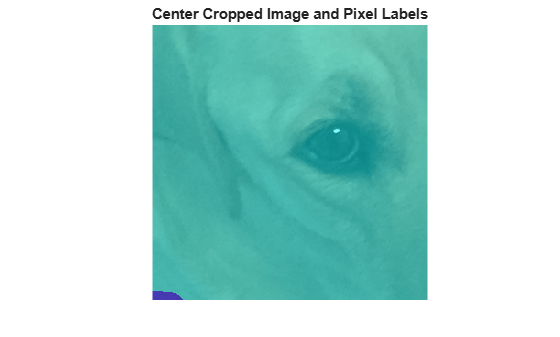Crop the image to the target size from a random position in the image.

```win = randomWindow2d(size(I),targetSize); croppedI = imcrop(I,win); croppedC = imcrop(C,win);```

Display the cropped labels over the cropped image.

```B = labeloverlay(croppedI,croppedC); imshow(B) title('Random Cropped Image and Pixel Labels')```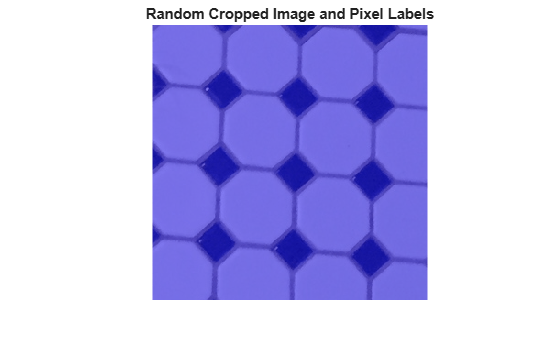### Warp Image and Pixel Labels

The `randomAffine2d` (Image Processing Toolbox) function creates a randomized 2-D affine transformation from a combination of rotation, translation, scaling (resizing), reflection, and shearing. Apply the transformation to images and pixel label images by using `imwarp` (Image Processing Toolbox). Control the spatial bounds and resolution of the warped output by using the `affineOutputView` (Image Processing Toolbox) function.

Rotate the input image and pixel label image by an angle selected randomly from the range [-50,50] degrees.

`tform = randomAffine2d("Rotation",[-50 50]);`

Create an output view for the warped image and pixel label image.

`rout = affineOutputView(size(I),tform);`

Use `imwarp` to rotate the image and pixel label image.

```rotatedI = imwarp(I,tform,'OutputView',rout); rotatedC = imwarp(C,tform,'OutputView',rout);```

Display the rotated labels over the rotated image.

```B = labeloverlay(rotatedI,rotatedC); imshow(B) title('Rotated Image and Pixel Labels')```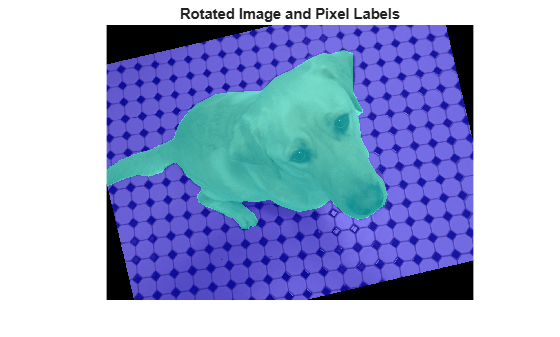### Apply Augmentation to Semantic Segmentation Training Data in Datastores

Datastores are a convenient way to read and augment collections of images. Create a datastore that stores image and pixel label image data, and augment the data with a series of multiple operations.

#### Create Datastores Containing Image and Pixel Label Image Data

To increase the size of the sample datastores, replicate the filenames of the image and pixel label image.

```numObservations = 4; trainImages = repelem({filenameImage},numObservations,1); trainLabels = repelem({filenameLabels},numObservations,1);```

Create an `imageDatastore` from the training image files. Create a `pixelLabelDatastore` from the training pixel label files. The datastores contain multiple copies of the same data.

```imds = imageDatastore(trainImages); pxds = pixelLabelDatastore(trainLabels,classes,ids);```

Associate the image and pixel label pairs by combining the image datastore and pixel label datastore.

`trainingData = combine(imds,pxds);`

Read the first image and its associated pixel label image from the combined datastore.

```data = read(trainingData); I = data{1}; C = data{2};```

Display the image and pixel label data.

```B = labeloverlay(I,C); imshow(B)```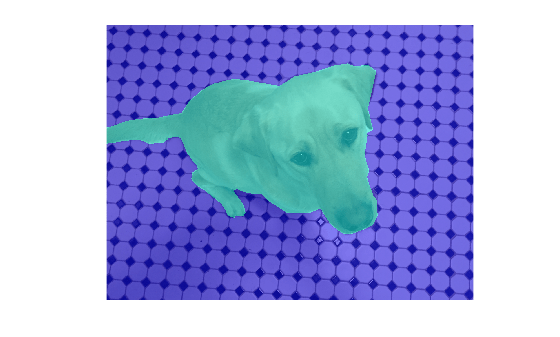#### Apply Data Augmentation

Apply data augmentation to the training data by using the `transform` function. This example performs two separate augmentations to the training data.

The first augmentation jitters the color of the image and then performs identical random scaling, horizontal reflection, and rotation on the image and pixel label image pairs. These operations are defined in the `jitterImageColorAndWarp` helper function at the end of this example.

`augmentedTrainingData = transform(trainingData,@jitterImageColorAndWarp);`

Read all the augmented data.

`data = readall(augmentedTrainingData);`

Display the augmented image and pixel label data.

```rgb = cell(numObservations,1); for k = 1:numObservations I = data{k,1}; C = data{k,2}; rgb{k} = labeloverlay(I,C); end montage(rgb)```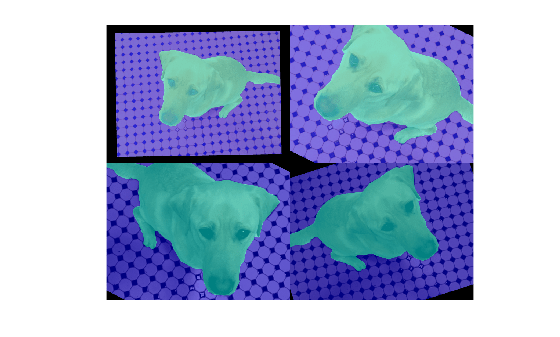The second augmentation center crops the image and pixel label image to a target size. These operations are defined in the `centerCropImageAndLabel` helper function at the end of this example.

```targetSize = [800 800]; preprocessedTrainingData = transform(augmentedTrainingData,... @(data)centerCropImageAndLabel(data,targetSize));```

Read all of the preprocessed data.

`data = readall(preprocessedTrainingData);`

Display the preprocessed image and pixel label data.

```rgb = cell(numObservations,1); for k = 1:numObservations I = data{k,1}; C = data{k,2}; rgb{k} = labeloverlay(I,C); end montage(rgb)```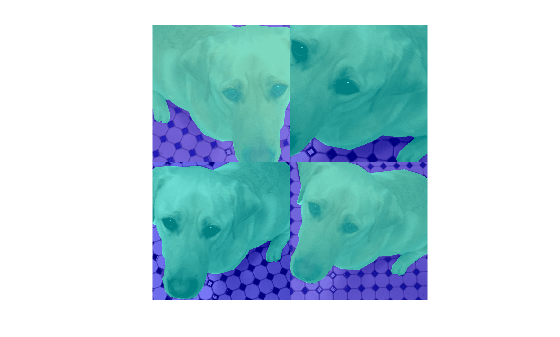#### Helper Functions for Augmentation

The `jitterImageColorAndWarp` helper function applies random color jitter to the image data, then applies an identical affine transformation to the image and pixel label image data. The transformation consists of a random combination of scaling by a scale factor in the range [0.8 1.5], horizontal reflection, and rotation in the range [-30, 30] degrees. The input `data` and output `out` are two-element cell arrays, where the first element is the image data and the second element is the pixel label image data.

```function out = jitterImageColorAndWarp(data) % Unpack original data. I = data{1}; C = data{2}; % Apply random color jitter. I = jitterColorHSV(I,"Brightness",0.3,"Contrast",0.4,"Saturation",0.2); % Define random affine transform. tform = randomAffine2d("Scale",[0.8 1.5],"XReflection",true,'Rotation',[-30 30]); rout = affineOutputView(size(I),tform); % Transform image and bounding box labels. augmentedImage = imwarp(I,tform,"OutputView",rout); augmentedLabel = imwarp(C,tform,"OutputView",rout); % Return augmented data. out = {augmentedImage,augmentedLabel}; end```

The `centerCropImageAndLabel` helper function creates a crop window centered on the image, then crops both the image and the pixel label image using the crop window. The input `data` and output `out` are two-element cell arrays, where the first element is the image data and the second element is the pixel label image data.

```function out = centerCropImageAndLabel(data,targetSize) win = centerCropWindow2d(size(data{1}),targetSize); out{1} = imcrop(data{1},win); out{2} = imcrop(data{2},win); end```